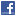## General Mathematics## MMM Imfinities Version 1.0 6 a4 Pages 29.09.2019) on Two Proposed Distinct Types of Imaginary (Im) Infinities ("imfinities") in Mathematics and Meta-Mathematics (Including Meta-Geometry), Emphasizing the Unlimited "Diversity" of Zero and Infinity

Authors: Andrei Lucian Dragoi

This paper proposes two distinct types of imaginary (im) infinities ("imfinities") in mathematics and meta-mathematics (including meta-geometry), emphasizing the unlimited "diversity" of zero and infinity, with far-reaching implications in all these domains, but also in math-related domains like modern physics, including the help in redefining the basics of Einstein's General relativity theory (GRT), quantum field theory (QFT), superstring theories (SSTs) and M-theory (MT). Keywords (including a list of main abbreviations): imaginary (im) infinities (“imfinities”), mathematics, metamathematics, metageometry, zero, infinity; Einstein’s General relativity theory (GRT); quantum field theory (QFT); superstring theories (SSTs); M-theory (MT);